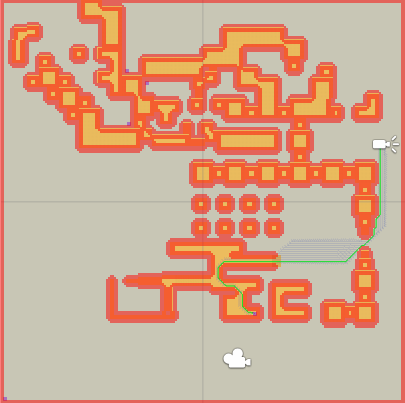A* Pathfinding Project  3.6 The A* Pathfinding Project for Unity 3D
Heuristic Optimization

A tutorial on how to use heuristic optimization to gain significant speedups

Normally when a pathfinding search is made, a heuristic is used. The heuristic is something which gives a rough estimate on how far it is at least to the target. Usually the distance to the target is used directly since this is fast and usually gives relatively good results. The problem is that in worlds which are not just open space with a few small obstacles scattered around, this estimate isn't very good. The result is that the search goes through a lot more nodes than it would have needed to if the heuristic had been better.

A* Pro Feature:
This is an A* Pathfinding Project Pro feature only. This function/class/variable might not exist in the Free version of the A* Pathfinding Project or the functionality might be limited
The Pro version can be bought here

If your world is static, or updates very rarely there is however a technique that can be used to precalculate distances between nodes and get heuristics which are a lot better. This can improve pathfinding performance by an order of magnitude (10x) in many cases.

The optimal heuristic is of course when we know the distance between every node to every other node in the graph, however this is a bit impractical since it would take a very long time to calculate and would use a lot of space.

So what can be done is that we select a few nodes (henceforth called "pivot points") and calculate the distances from those nodes to every other node in the graph. This can then be used to calculate pretty good heuristics.

Regardless of what heuristic is used, the A* algorithm will always search through all nodes which could lie on the shortest path(s) so this technique will for example not give any performance increase on large open grid graphs because a path search rarely searches much more than the nodes on the shortest paths anyway.

The image below shows a completely empty grid graph and the nodes a single path request searched through. All those nodes lie on the optimal path(s) because in a grid, there are many optimal paths.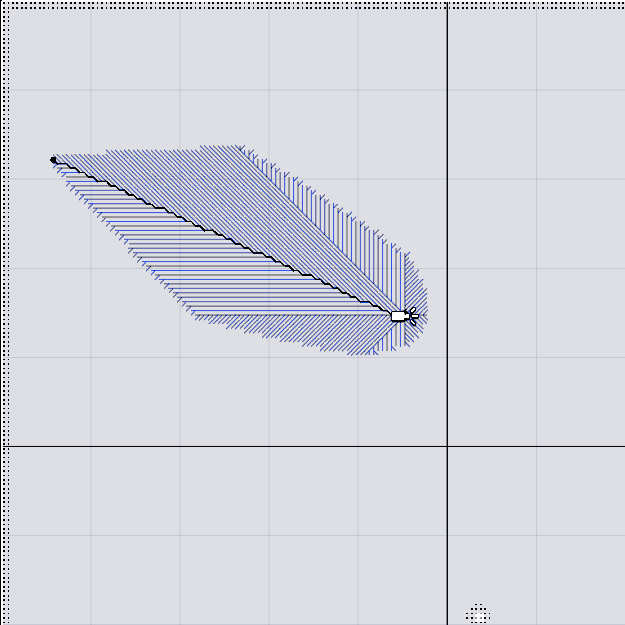The A* Pathfinding Project has algorithms for automatically selecting pretty good pivot points because it can be quite hard to select them in a good way manually. When I profile, I sometimes find that my manual placements of pivot points is worse than just randomly selected points.

# Placing Pivot Points Manually

When placing these pivot points, the key thing about them is that they should be at dead ends in the world. The perform best when many paths can be extended to the pivot point and still be a shortest path.

The the image below you can see pivot points in purple. The agent in the bottom left corner has requested a path to the green circle.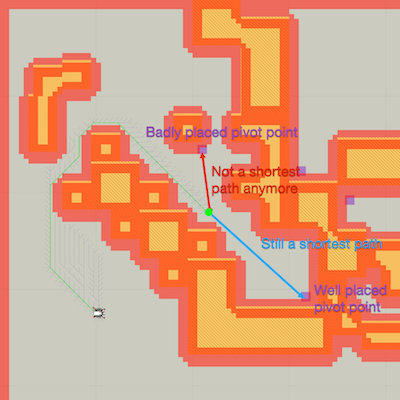There can also be game specific knowledge you can apply. For example in a TD game, almost all units will move towards a single goal. So if you just place a single pivot point at the goal all paths will be much faster to calculate because the optimal distance to that point from every other one is already known.

# Placing Pivot Points Automatically

Two modes exist. Either all points are chosen randomly or an algorithm is applied which selects nodes as far away from each other as possible. The second one gives much higher quality heristics, but it is much slower to calculate as it requires that the inital distance calculation is done in serial and multithreading is therefore of no use.

In the image below a comparison is shown between 5 randomly selected points and 5 points far away from each other. The ones which are far away from each other tend to be placed in dead ends or in the corners of the map, which will give very good results.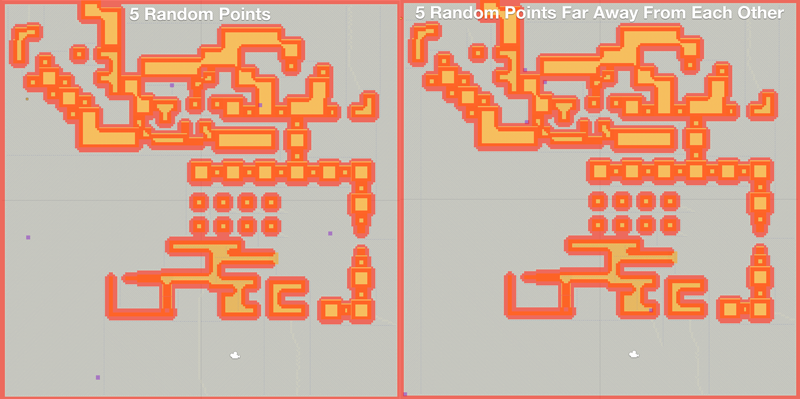The number of pivot points that you should use varies. The best solution is just to try different values and see what is best for your game. When reaching over a hundred pivot points the overhead from them is often greater than the gain from a reduced number of nodes to search. I would recommend between 1 and 100, try different values and see what works best.

You might notice that there is a delay at the start of the game until the pathfinding starts working. This is because it is busy preprocessing the heuristic lookups. If you find this delay so large that it becomes a problem, switch to using the "Random" mode instead of the "Random Spread Out" (if you were using that) or decreasing the number of pivot points.

# Results

Heuristic optimization disabled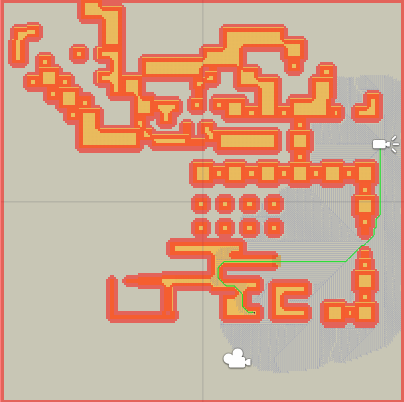Heuristic optimization enabled